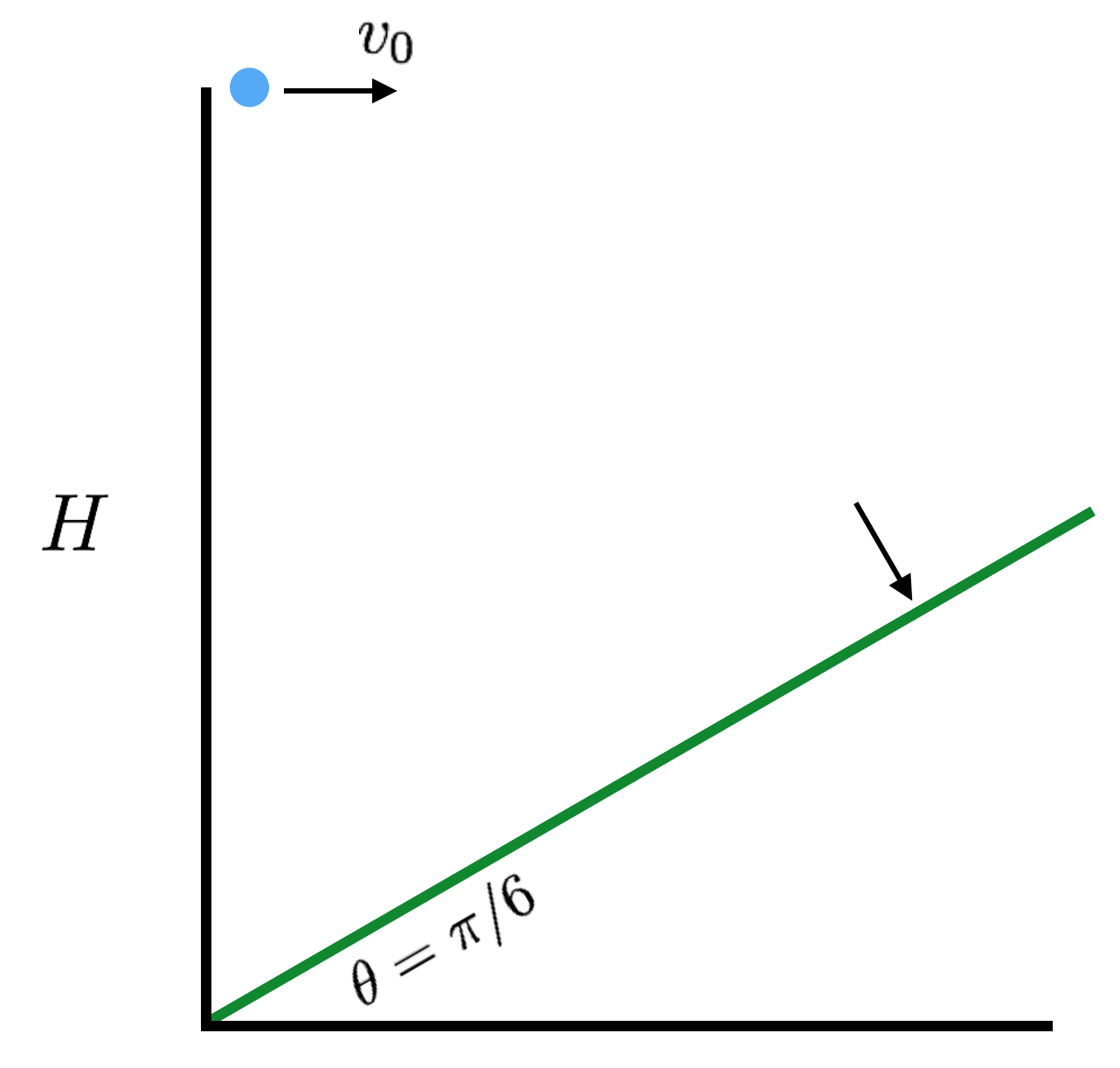# Hit The Right AngleA particle is projected with velocity $v_0$ horizontally from a height $H$ over a hill, and strikes the hill perpendicularly.

Find $2gH/v_0 ^ 2$

Assume the hill rises linearly from the origin at an angle $\theta = \dfrac\pi6 = \si{30}^\circ$.

×